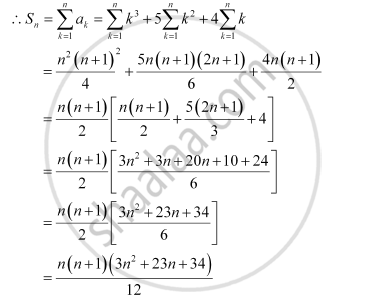Share

# Find the Sum to N Terms of the Series Whose Nth Term is Given by N (N + 1) (N + 4). - Mathematics

#### Question

Find the sum to n terms of the series whose nth term is given by n (n + 1) (n + 4).

#### Solution

an = n (n + 1) (n + 4) = n(n+ 5n + 4) = n3 + 5n2 + 4nIs there an error in this question or solution?